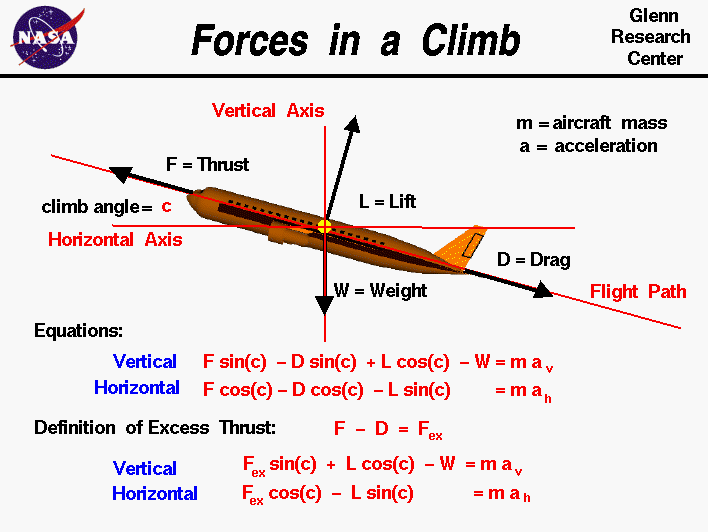PRINT THIS ANSWER SHEET TO FILL OUT AND TURN IN.

NAME_________________________________ CLASS____________________ DATE____________

### Beginner's Guide to Propulsion

Forces in a Climb - Vectors - Worksheet
1. Using the first equation: L cos (c) + F sin (c) -D sin (c) - W = ma (vertical)
and the third equation: F -D = Fex , explain and show how to obtain
the fourth equation: L cos (c) + Fex sin (c) - W = ma (vertical).

2. Using the second equation and the third equation, explain and show how to obtain the fourth equation.

3.  Why isn't W multiplied by a sine or cosine?

4. Using the given diagram, theorems/postulates of geometry, and vectors, explain each term in the first two equations.